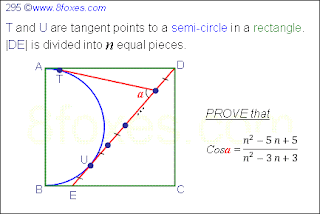## Sunday, June 27, 2010

### Fox 295Let the one who lives, live with an evidence,
Let the one who dies, perish with an evidence.

#### 1 comment:

1.Following the the same kind of reasoning as b4, DU=AD=BC=nx-x and the tangents at T&U are=nx-2x. Further, DC^2=(nx)^2-(nx-2x)^2 or DC=2x√(n-1). So tan(α/2) =x√(n-1)/x(n-2)=√(n-1)/(n-2) or (sec(α/2))^2= 1+(n-1)/(n-2)^2 =(n^2-4n+4+n-1)/(n-2)^2 =(n^2-3n+3)/(n^2-4n+4) and hence (cos(α/2))^2=(n^2-4n+4)/(n^2-3n+3) or cos(α)= 2(n^2-4n+4)/(n^2-3n+3)-1=(n^2-5n+5)/(n^2-3n+3)